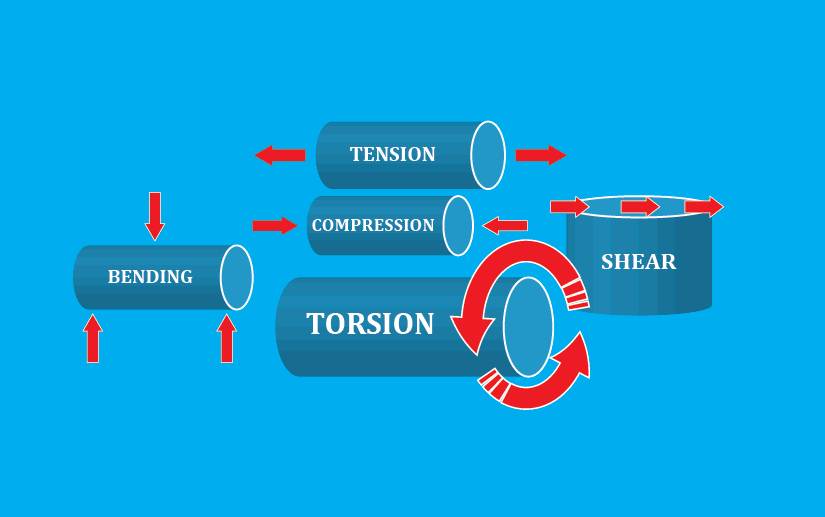Modified: June 16, 2021

# Stresses in Machine Elements

When designing new machines, stress analysis is one of the major factors that design engineers consider. Otherwise, the machine is more likely to be fail. However, the most non-engineers, do not really care about calculations. And they design the product with higher assumed values. However, in the economical perspective, it’s not that effective.

Simply, stress is a force acting on an unit area. In other words, the stress is the force divided by area.

Stress is represented in few symbols, and the most common usage is lower case Greek letter Sigma – σ. However, in many engineering textbooks, it’s represented as lower case Greek letter Tau – τ.

In this article, you’ll learn different types of stresses act on machine elements/ parts.

## Tensile Stress

In simple words, tensile stress is the resistance of a material to tear in to parts. If we pull a cylindrical object in two sides, the stress is the amount of force applied, divided by the area of the base of the base of the cylinder.

When we measure the stress, along with the change in length (strain), we can see few noticeable changes in stress. And this curve is known as stress-strain curve.

These noticeable changes in the stress, are knows as strength of points. Strength is a point that something of interest occurs. In other words, it’s the capacity of a material to take stress.

In stress-strain curve, we can meet three stresses.

1. Yield Strength
2. Ultimate Tensile Strength
3. Fracture Strength

## Compressive stress

In simple words, compressive stress is the resistance of a material compress. For example, if you push a cylindrical object in two opposite directions, the compressive stress is the amount of force applied divided by the area of base of the cylinder.

Lets consider the image below. In this image, the metallic specimen is subjected to a resultant force acting along the axis of the cylinder. And the force is acting on a cross-sectional area perpendicular to the base.

## Shear stress

Shear stress is the resistance to deform due to perpendicular forces acting along with the surface. For example, if you try to push your desk, parallel and along with the desk surface, the shear stress is equal to the amount of force applied, divided by the area.

A force which is along with the surface and perpendicular to the surface is known tangential force. So, we can say that when a body is subjected to two equal forces in opposite direction,

In other words, when a body is subjected to two equal and tangential forces acting on a object in opposite directions, the body try to shear off the section, and the stress induced is the shear stress.

## Bending Stress

In simple words, bending stress is the resistance of an object to bend. In bending the force is a normal load and applied at a particular point of the object.

The basic principal of bending stress is when the top layer is under compression and the bottom layer is under tension. In between these layers, there is an neutral stretched layer which hasn’t subjected to a stress.

Unlike in other stresses we talked earlier, bending stress is not calculated by simply dividing the stress by area. Therefore, to calculate bending stress, we have to use the bending moment, vertical distance away from the neutral axis and moment of inertia around the neutral axis.

Bending moment is a measure of bending effect which is developed internally due to external loads. So, the moment developed is balanced by the moment developed. To calculate the bending moment of a point, we multiply the force acting on the structure and the perpendicular distance to the point.

## Torsional Stress

In simple words, torsional stress a the shear stress occurred due to twisting. Or can call it the angular deformation of a body.

Torsional stress is one of the complicated stresses. To calculate the torsional stress, we have to build a relationship torque and the shear stress.